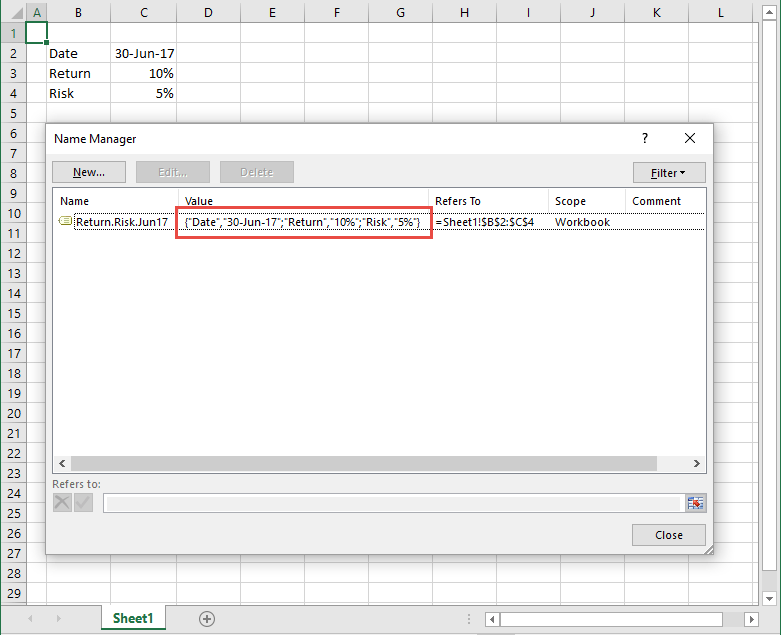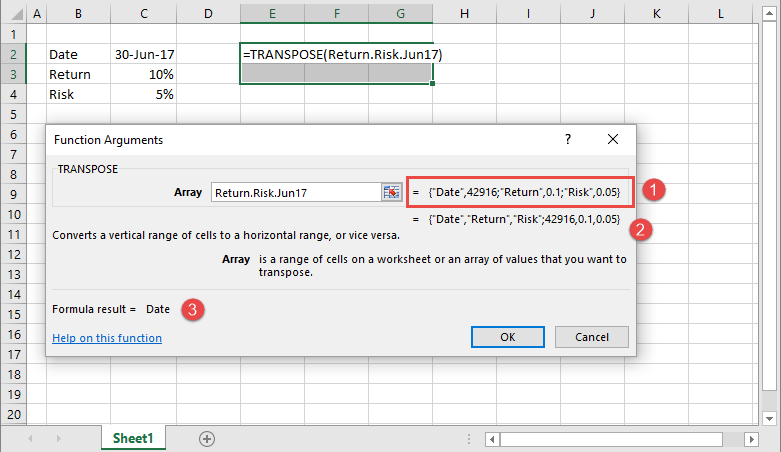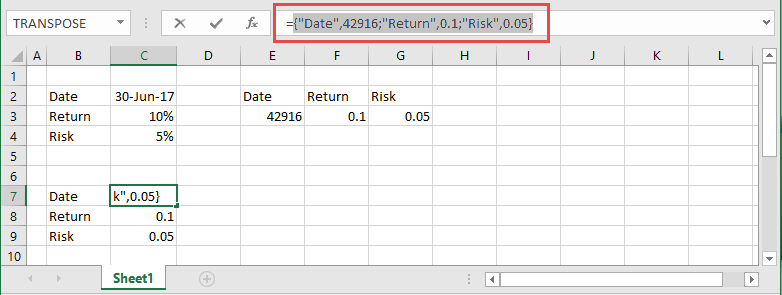# Arrays (1)

## 1. An array

In an Excel worksheet, an array is a rectangular range of cells described by its dimension. Dimension is the number of rows (m), and number of columns (n). The number of rows is first, followed by the number of columns. For example, a 3 x 4 array (pronounced 3 by 4) has 3 rows and 4 columns. It is displayed as 3R x 4C in Excel.

Arrays are usually passed to, or returned by a formula or function.

• A column vector - is an array with one column and multiple rows. An m x 1 array
• A row vector - is an array with one row and multiple columns. A 1 x n array
• A multidimensional array - is an m x n array with multiple rows and multiple columns

Excel arrays are contained within a pair of curly braces. For example, {1,2,3,4} is a row vector with 4 elements (4 columns) ie. a 1 x 4 array. In applications such as Matlab, square brackets are used, [1,2,3,4].

## 2. Element-by-element operations

To perform element by element operations, each array must be of the same dimension, ie. the same number of rows and columns.

Example: A = {1,2,3,4}, and B = {5,6,7,8} are row vectors

Multiplication

A x B = {5,12,21,32}. This is equivalent to 1 x 5, 2 x 6, 3 x 7, 4 x 8

Division

A / B = {0.2,0.333333333,0.428571429,0.5}. This is equivalent to 1 / 5, 2 / 6, 3 / 7, 4 / 8

Example: C = {1;2;3;4}, D = {5;6;7;8}

C + D = {6,8,10,12}. This is equivalent to 1 + 5, 2 + 5, 3 + 7, 4 + 8

Subtraction

D - C = {6,8,10,12}. This is equivalent to 5 -1,

### 2.1 Functions that include array arguments

• FREQUENCY(data_array, bins_array)
• HLOOKUP(lookup_value, table_array, row_index_num, [range_lookup])
• INDEX(array, row_num, [column_num])
• LOOKUP(lookup_value, array)
• MATCH(lookup_value, lookup_array, [match_type])
• MODE.MULT((number1,[number2],...) Note: Returns a vertical array
• MDETERM(array) Note: requires the CSE sequence
• MINVERSE(array) Note: requires the CSE sequence
• MMULT(array1, array2) Note: requires the CSE sequence
• SUMPRODUCT(array1, [array2], [array3], ...)
• SUMX2MY2(array_x, array_y)
• SUMX2PY2(array_x, array_y)
• SUMXMY2(array_x, array_y)
• TRANSPOSE(array) Note: requires the CSE sequence
• VLOOKUP (lookup_value, table_array, col_index_num, [range_lookup])Fig 1: Name manager - showing the Value property of the Return.Risk.2017 defined name in array formatFig 2: Function arguments dialog box - showing the source array, the result array for CSE, and the result array for Enter (OK)Fig 3: Formula bar - the formula is =Return.Risk.2017. Pressing F2, selecting the array name, then F9 displays the array / reference contents

### What is the dimension of the array?

By using the size of the selection. To determine the number of rows and columns:

1. Use the address coordinates of the range.
2. Navigating the selection
• left click and drag - dimension appears in the Name Box
• if the selection requires scrolling of the screen, the dimension appears near the fill handle, whilst the left button is depressed
• use a macro such as GetSelectDim
• Select the Current Region (Ctrl + A) then extend the selection one row (Shift + ) or one column (Shift + ), then revert back (Shift + in the case of a column). The Active Cell must the left column or top row.

• Published: 23 July 2015
• Revised: Tuesday 25th of February 2020 - 01:37 PM, [Australian Eastern Standard Time (EST)]